# Exponents Chapter Exam

Exam Instructions:

Choose your answers to the questions and click 'Next' to see the next set of questions. You can skip questions if you would like and come back to them later with the yellow "Go To First Skipped Question" button. When you have completed the practice exam, a green submit button will appear. Click it to see your results. Good luck!

### Page 1

#### Question 1 1. Simplify the expression using positive exponents.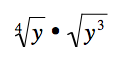#### Question 2 2. Simplify the following expression using positive exponents only.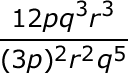#### Question 3 3. Which of the following is equivalent to the following expression?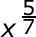#### Question 4 4. Simplify the expression using positive exponents.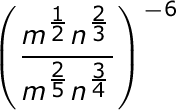#### Question 5 5. Simplify using ONLY positive exponents.### Page 2

#### Question 6 6. Simplify the expression using positive exponents.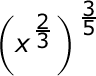#### Question 7 7. Which of the following radical expressions is equal to this exponential expression?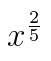#### Question 8 8. Which of the following would be true regarding the following exponential expression?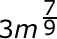#### Question 9 9. Simplify using only positive exponents.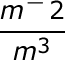#### Question 10 10. Simplify the expression using positive exponents.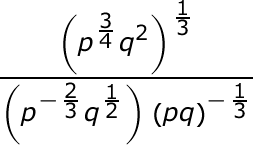### Page 3

#### Question 11 11. Simplify the expression below.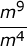#### Question 12 12. Which of the following is equivalent to the expression below?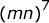#### Question 13 13. Which of the following is equivalent to the expression below?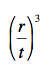#### Question 14 14. Simplify the expression using positive exponents.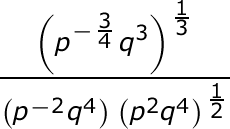#### Question 15 15. Which of the following is equivalent to the expression below?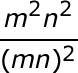### Page 4

#### Question 17 17. Which of the following is equivalent to the following expression?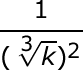#### Question 18 18. Simplify the expression below using only positive exponents.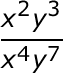#### Question 19 19. Simplify the following expression using positive exponents.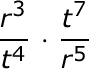#### Question 20 20. Simplify the following expression.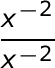### Page 5

#### Question 21 21. Simplify the following expression.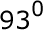#### Question 22 22. Simplify the expression below using positive exponents only.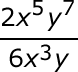#### Question 23 23. Simplify using ONLY positive exponents.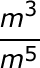#### Question 24 24. Which of the following exponential expressions is equal to this radical expression?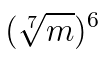#### Question 25 25. Which of the following is equivalent to the expression below?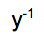#### Exponents Chapter Exam Instructions

Choose your answers to the questions and click 'Next' to see the next set of questions. You can skip questions if you would like and come back to them later with the yellow "Go To First Skipped Question" button. When you have completed the practice exam, a green submit button will appear. Click it to see your results. Good luck!

Support